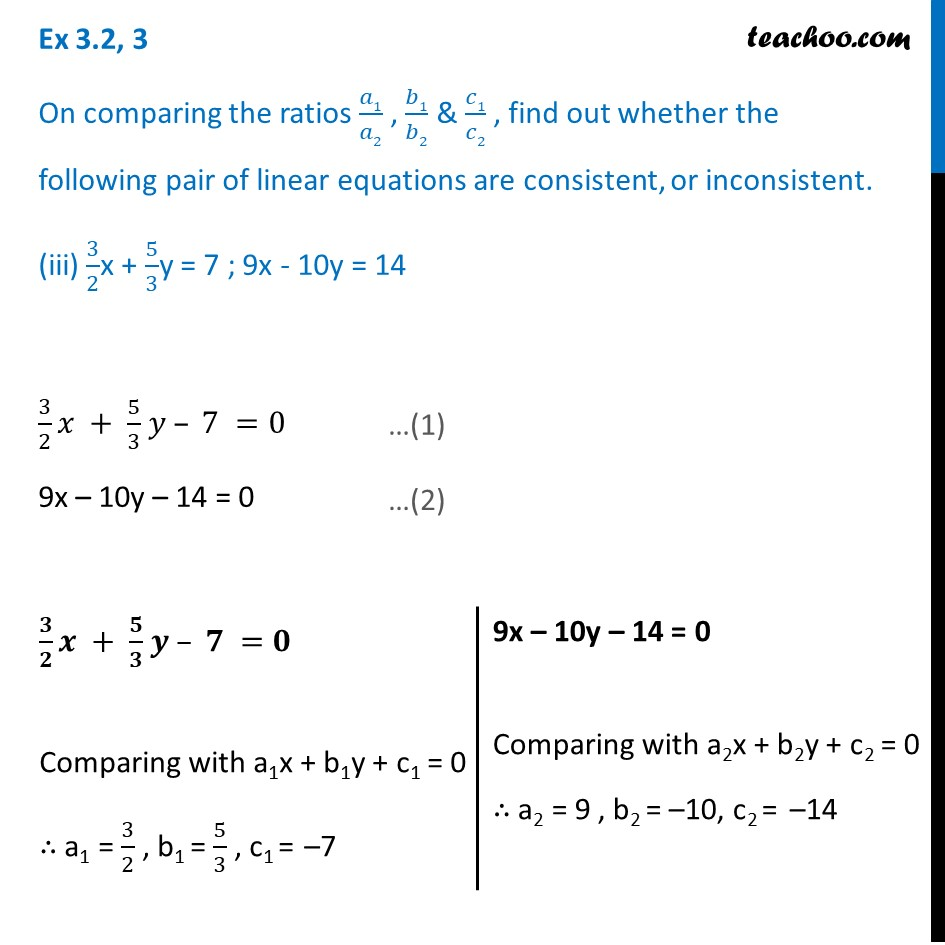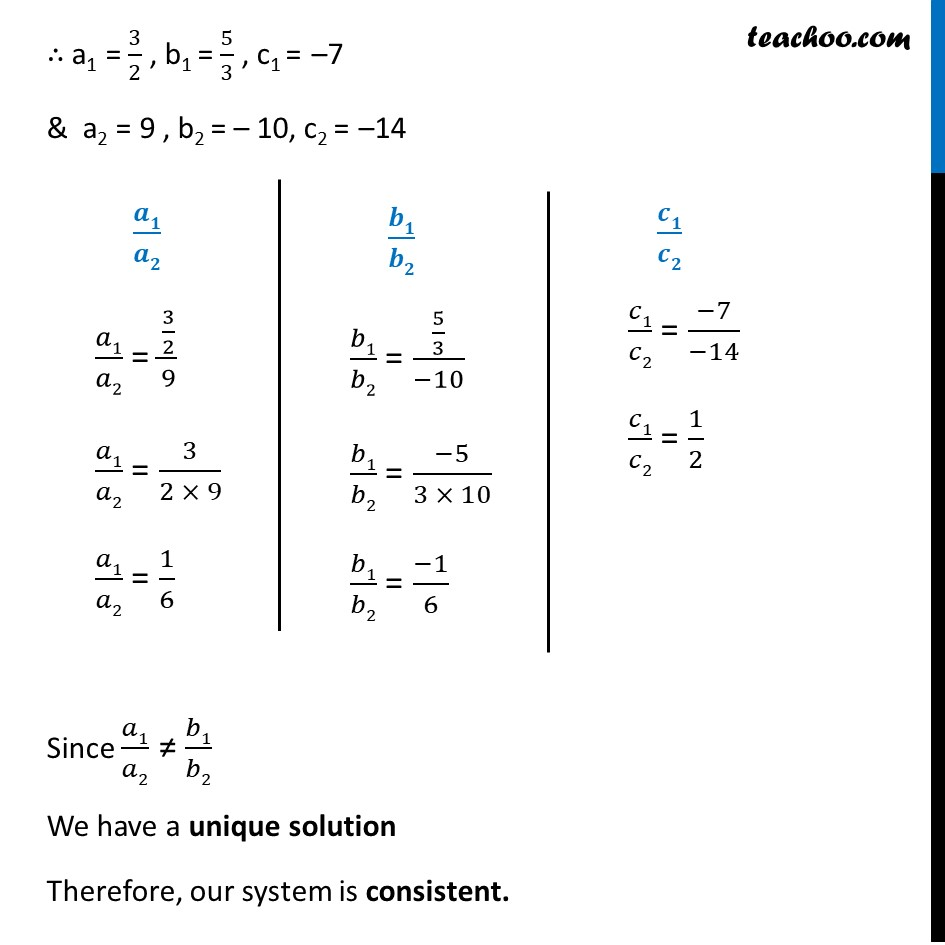1. Chapter 3 Class 10 Pair of Linear Equations in Two Variables (Term 1)
2. Serial order wise
3. Ex 3.2

Transcript

Ex 3.2, 3 On comparing the ratios 𝑎1/𝑎2 , 𝑏1/𝑏2 & 𝑐1/𝑐2 , find out whether the following pair of linear equations are consistent, or inconsistent. (iii) 3/2x + 5/3y = 7 ; 9x - 10y = 14 3/2 𝑥 + 5/3 𝑦 – 7 =0 9x – 10y – 14 = 0 𝟑/𝟐 𝒙 + 𝟓/𝟑 𝒚 – 𝟕 =𝟎 Comparing with a1x + b1y + c1 = 0 ∴ a1 = 3/2 , b1 = 5/3 , c1 = –7 9x – 10y – 14 = 0 Comparing with a2x + b2y + c2 = 0 ∴ a2 = 9 , b2 = –10, c2 = –14 ∴ a1 = 3/2 , b1 = 5/3 , c1 = –7 & a2 = 9 , b2 = – 10, c2 = –14 𝒂𝟏/𝒂𝟐 𝑎1/𝑎2 = (3/2)/9 𝑎1/𝑎2 = 3/(2 × 9) 𝑎1/𝑎2 = 1/6 𝒃𝟏/𝒃𝟐 𝑏1/𝑏2 = (5/3)/(−10) 𝑏1/𝑏2 = (−5)/(3 × 10) 𝑏1/𝑏2 = (−1)/6 𝒄𝟏/𝒄𝟐 𝑐1/𝑐2 = (−7)/(−14) 𝑐1/𝑐2 = 1/2 Since 𝑎1/𝑎2 ≠ 𝑏1/𝑏2 We have a unique solution Therefore, our system is consistent.